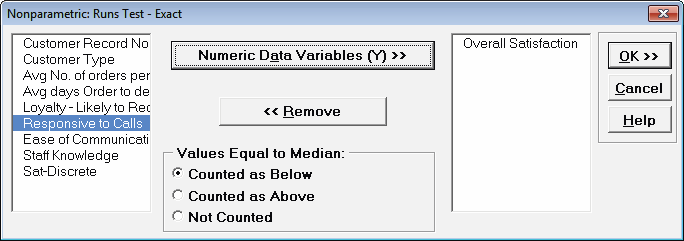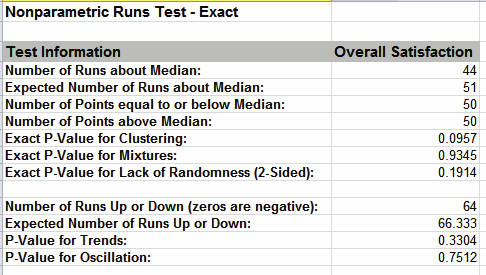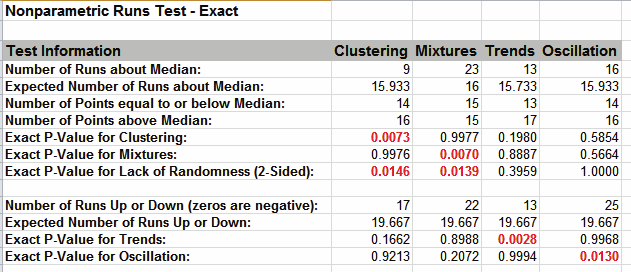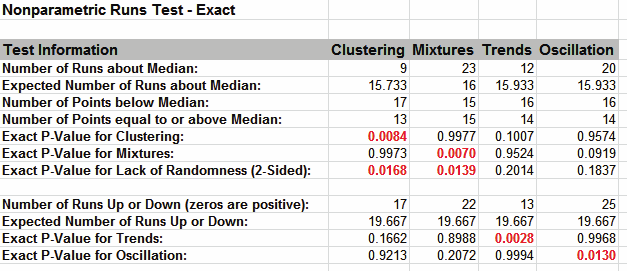Include Top

# Nonparametric Runs Test for Randomness - Exact

The Nonparametric Runs test does not assume that the sample data are normally distributed, but it does assume that the test statistic follows a Normal distribution when computing the “large sample” or “asymptotic” P-Value. With a small sample size (N <= 50), this approximation may be invalid, so exact methods should be used. SigmaXL computes the exact P-Values utilizing permutations.

It is important to note that while exact P-Values are “correct,” they do not increase (or decrease) the power of a small sample test, so they are not a solution to the problem of failure to detect a trend due to inadequate sample size.

Clustering, Mixtures and Lack of Randomness (Runs Above/Below)

If Count (N) is greater than 1000, the exact P-Value is estimated using a continuity-corrected normal approximation. Since the Runs Test Exact P-Value is computed very quickly for sample sizes as large as 1000, Monte Carlo P-Values are not required.

Trends and Oscillation (Runs Up/Down)

Exact P-Values are derived from published tables, given the sample size and the number of up/down runs. The exact tables apply to N <= 50. If N > 50, a continuity-corrected normal approximation is used.

Exact P-Values are not available in Run Charts.

For further details and references refer to the Appendix Exact and Monte Carlo P-Values for Nonparametric and Contingency Tests.

We will now redo the Customer Data Overall Satisfaction example to compute exact P-Values. Typically this would not be necessary unless the sample sizes were smaller (N <= 50), but this gives us continuity on the example. We will also consider the above small sample examples later.

1. Open Customer Data.xlsx, click Sheet 1 tab.
2. Click SigmaXL > Statistical Tools > Nonparametric Tests – Exact > Runs Test – Exact. If necessary, check Use Entire Data Table, click Next.
3. Select Overall Satisfaction, click Numeric Data Variables (Y) >>. Set Values Equal to Median: to Counted as Below.Note on Options for Clustering, Mixtures and Lack of Randomness (Runs Above/Below)

If none of the observations are equal to the sample median, the options for “Values Equal to Median:” do not affect the exact P-Value. That is the case with this Customer Data example. In cases where there are observations equal to the sample median, then the exact P-Value will be different for each option selected. For regular Runs Test, SigmaXL uses Counted as Below (in agreement with Minitab). StatXact uses Counted as Above for the Runs Test. Matlab uses Not Counted. Users should try Counted as Below and Counted as Above to ensure that the P-Values agree on reject or fail to reject H0.

Note on Options for Trends and Oscillation (Runs Up/Down)

The Values Equal to Median options also apply to the Runs Up/Down Test: zeros of the first order differences (i.e., two consecutive values that are the same). Counted as Below denotes “counted as negative.” Counted as Above denotes “counted as positive.” Not Counted denotes that zero first order differences are deleted. This consolidation of options was done to keep the dialog simple.

Missing values are ignored for all exact runs tests.

Use of these options will be demonstrated in the small sample example.

4. Click OK. Resulting Output:

5.With all of the P-Values being greater than 0.01 (alpha = .01 is preferred for the Runs Test to minimize false alarms), we fail to reject H0, and conclude that the data is random (or statistically independent).

The Above/Below and Up/Down Runs counts are identical to the above “large sample” or “asymptotic” results. The Clustering/Mixtures/Randomness (Above/Below) Exact P-Values are close but slightly different. This was expected because the sample size is reasonable (N > 50), so the “large sample” P-Values are valid using a normal approximation.

The P-Values for Trends and Oscillation (Up/Down) use a normal approximation because the sample size is greater than 50. They are, however, slightly different than the “large sample” above because a continuity correction is now applied to the normal approximation.

6. Now we will consider the small sample examples. Open Runs Test Example Data.xlsx, click Runs Test Example Data tab.
7. Click SigmaXL > Statistical Tools > Nonparametric Tests – Exact > Runs Test – Exact. If necessary, check Use Entire Data Table, click Next.
8. Select Clustering and shift click to Oscillation, click Numeric Data Variables (Y) >>. Set Values Equal to Median: to Counted as Below.9. Click OK. Resulting output:The Exact P-Values are close to the above “large sample” or “asymptotic” results, but note that some of the values are now greater than .01 so they would fail-to reject H0. Note that this does not imply that the large sample runs test is more powerful, but rather we cannot conclude some of the previously identified patterns at the 99% confidence level.

We will now rerun the analysis using the Counted as Above option.

10. Press F3 or click Recall SigmaXL Dialog to recall last dialog. Set Values Equal to Median: to Counted as Above.11. Click OK. Results:Note the change in Trends and Oscillation Number of Runs and resulting dramatic change to the Exact P-Values.

In conclusion, when using Runs Test Exact, always try Counted As Below and Counted as Above to ensure that the P-Values agree with each other. Also, whenever you have a small sample size and are performing a Nonparametric test, always use Exact.

# Web Demos

Our CTO and Co-Founder, John Noguera, regularly hosts free Web Demos featuring SigmaXL and DiscoverSim